### Tests Based on the Empirical Distribution Function

If you specify the EDF option, PROC NPAR1WAY computes tests based on the empirical distribution function. These include the Kolmogorov-Smirnov and Cramer-von Mises tests, and also the Kuiper test for two-sample data. This section gives formulas for these test statistics. For further information about the formulas and the interpretation of EDF statistics, see Hollander and Wolfe (1999) and Gibbons and Chakraborti (1992). For details about the-sample analogs of the Kolmogorov-Smirnov and Cramer-von Mises statistics, see Kiefer (1959).

The empirical distribution function (EDF) of a sample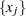,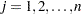, is defined as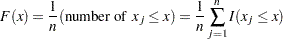where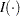is an indicator function. PROC NPAR1WAY uses the subsample of values within theth class level to generate an EDF for the class,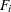. The EDF for the overall sample, pooled over classes, can also be expressed as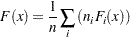where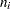is the number of observations in theth class level, andis the total number of observations.

#### Kolmogorov-Smirnov Test

The Kolmogorov-Smirnov statistic measures the maximum deviation of the EDF within the classes from the pooled EDF. PROC NPAR1WAY computes the Kolmogorov-Smirnov statistic as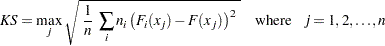The asymptotic Kolmogorov-Smirnov statistic is computed as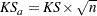For each class leveland overall, PROC NPAR1WAY displays the value ofat the maximum deviation fromand the value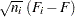at the maximum deviation from. PROC NPAR1WAY also gives the observation where the maximum deviation occurs.

If there are only two class levels, PROC NPAR1WAY computes the two-sample Kolmogorov-Smirnov test statistic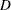as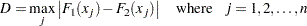The p-value for this test is the probability thatis greater than the observed value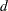under the null hypothesis of no difference between class levels (samples). PROC NPAR1WAY computes the asymptotic p-value forwith the approximation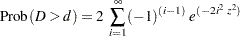where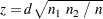The quality of this approximation has been studied by Hodges (1957).

If you specify the D option, or if you request exact Kolmogorov-Smirnov p-values with the KS option in the EXACT statement, PROC NPAR1WAY also computes the one-sided Kolmogorov-Smirnov statistics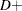and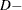for two-sample data as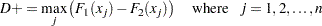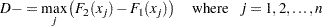The asymptotic probability thatis greater than the observed value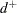, under the null hypothesis of no difference between the two class levels, is computed as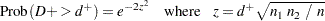Similarly, the asymptotic probability thatis greater than the observed value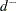is computed as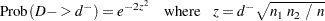To request exact p-values for the Kolmogorov-Smirnov statistics, you can specify the KS option in the EXACT statement. See the section Exact Tests for more information.

#### Cramer-von Mises Test

The Cramer-von Mises statistic is defined as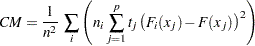where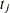is the number of ties at theth distinct value andis the number of distinct values. The asymptotic value is computed as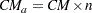PROC NPAR1WAY displays the contribution of each class level to the sum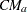.

#### Kuiper Test

For data with two class levels, PROC NPAR1WAY computes the Kuiper statistic, its scaled value for the asymptotic distribution, and the asymptotic p-value. The Kuiper statistic is computed as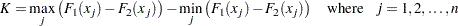The asymptotic value isPROC NPAR1WAY displays the value of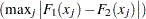for each class level.

The p-value for the Kuiper test is the probability of observing a larger value of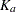under the null hypothesis of no difference between the two classes. PROC NPAR1WAY computes this p-value according to Owen (1962, p. 441).